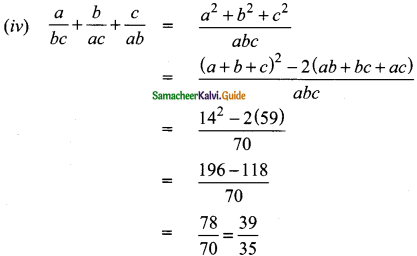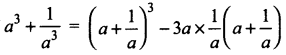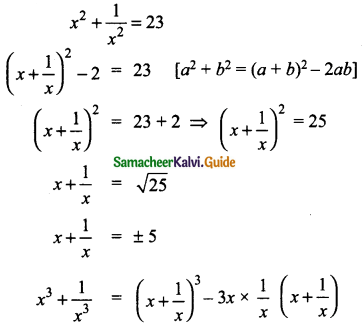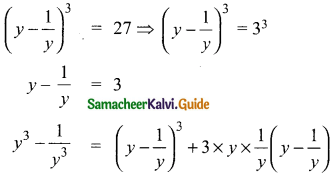Students can download Maths Chapter 3 Algebra Ex 3.4 Questions and Answers, Notes, Samacheer Kalvi 9th Maths Guide Pdf helps you to revise the complete Tamilnadu State Board New Syllabus, helps students complete homework assignments and to score high marks in board exams.

## Tamilnadu Samacheer Kalvi 9th Maths Solutions Chapter 3 Algebra Ex 3.4

Question 1.
Expand the following:
(i) (2x + 3y + 4z)2
(ii) (-p + 2q + 3r)2
(iii) (2p + 3) (2p – 4) (2p – 5)
(iv) (3a + 1) (3a – 2) (3a + 4)
Solution:
We know that (a + b + c)2 = a2 + b2 + c2 + 2ab + 2bc + 2ac
(i) (2x + 3y + 4z)2 = (2x)2 + (3y)2 + (4z)2 + 2(2x) (3y) + 2(3y) (4z) + 2(4z) (2x)
= 4x2 + 9y2 + 16z2 + 12xy + 24yz + 16xz

(ii) (-p + 2q + 3r)2 = (-p)2 + (2q)2 + (3r)2 + 2(-p) (2q) + 2(2q)(3r) + 2(3r) (- p)
= p2+ 4q2 + 9r2 – 4pq + 12qr – 6pr(iii) (2p + 3) (2p – 4) (2p – 5)
[Here x = 2p, a = 3, b = -4 and c = -5]
= (2p)3 + (3 – 4 – 5) (2p)2 + [(3)(-4) + (-4)(-5) + (3) (-5)] 2p + (3) (-4) (-5)
= 8p3 + (-6)(4p2) + (-12 + 20 – 15) 2p + 60
= 8p3 – 24p2 – 14p + 60

(iv) (3a + 1) (3a – 2) (3a + 4)
[Here x = 3a, a = 1, b = -2 and c = 4]
= (3a)3 + (1 – 2 + 4) (3a)2 + [(1)(-2) + (-2) (4) + (4) (1)] (3a) + (1) (-2) (4)
= 27a3 + 3(9a2) + (-2 – 8 + 4) (3a) – 8
= 27a3 + 27a2 – 18a – 8Question 2.
Using algebraic identity, find the coefficients of x2, x and constant term without actual expansion.
(i) (x + 5)(x + 6)(x + 7)
Solution:
[Here x = x, a = 5, b = 6, c = 7]
(x + a) (x + b) (x + c) = x3 + (a + b + c)x2 + (ab + bc + ac)x + abc
coefficient of x2 = 5 + 6 + 7
= 18
coefficient of x = 30 + 42 + 35
= 107
constant term = (5) (6) (7)
= 210(ii) (2x + 3)(2x – 5) (2x – 6)
Solution:
[Here x = 2x, a = 3, b = -5, c = -6]
(x + a) (x + b) (x + c) = x3 + (a + b + c)x2 + (ab + bc + ac)x + abc
coefficient of x2 = (3 – 5 – 6)4 [(2x)2 = 4x2]
= (-8) (4)
= -32
coefficient of x = [(3)(-5) + (-5)(-6) + (-6)(3)](2)
= (-15 + 30-18) (2)
= (-3) (2)
= -6
constant term = (3) (-5) (-6)
= 90Question 3.
If (x + a)(x + b)(x + c) = x3 + 14x2 + 59x + 70, find the value of
(i) a + b + c
(ii) $$\frac{1}{a} + \frac{1}{b} + \frac{1}{c}$$
(iii) a2 + b2 + c2
(iv) $$\frac{a}{bc} + \frac{b}{ac} + \frac{c}{ab}$$
Solution:
(x + a) (x + b) (x + c) = x3 + 14x2 + 59x + 70
x3 + (a + b + c)x2 + (ab + bc + ac)x + abc = x3 + 14x2 + 59x + 70
a + b + c = 14, ab + bc + ac = 59, abc = 70
(i) a + b + c = 14

(ii) $$\frac{1}{a} + \frac{1}{b} + \frac{1}{c}$$ = $$\frac{bc+ac+ab}{abc}$$
= $$\frac{59}{70}$$

(iii) a2 + b2 + c2 = (a + b + c)2 – 2 (ab + bc + ac)
= (14)2 – 2(59)
= 196 – 118
= 78Question 4.
Expand:
(i) (3a – 4b)3
Solution:
(a – b)3 = a3 – b3 – 3ab (a – b)
(3a – 4b)3 = (3a)3 – (4b)3 – 3(3a)(4b)(3a – 4b)
= 27a3 – 64b3 – 36ab (3a – 4b)
= 27a3 – 64b3 – 108a2b + 144ab2

(ii) [x + $$\frac{1}{y}]^{3}$$
Solution:
(a + b)3 = a3 + b3 + 3ab (a + b)Question 5.
Evaluate the following by using identities:
(i) 983
Solution:
983 = (100 – 2)3 [(a – b)3 = a3 – b3 – 3ab (a – b)]
= 1003 – (2)3 – 3(100) (2) (100 – 2)
= 1000000 – 8 – 600(98)
= 1000000 – 8 – 58800
= 1000000 – 58808
= 941192

(ii) 10013
Solution:
(1001)3 = (1000 + 1)3
[(a + b)3 = a3 + b3 + 3ab (a + b)]
= (1000)3 + 13 + 3(1000) (1) (1000 + 1)
= 1000000000 + 1 + 3000 (1001)
= 1000000001 + 3003000
= 1003003001Question 6.
If (x + y + z) = 9 and (xy + yz + zx) = 26, then find the value of x2 + y2 + z2.
Solution:
x + y + z = 9; xy + yz + zx = 26
x2 + y2 + z2 = (x + y + z)2 – 2xy – 2yz – 2xz
= (x + y + z)2 – 2 (xy + yz + zx)
= 92 – 2(26)
= 81 – 52
= 29

Question 7.
Find 27a3 + 64b3, If 3a + 4b = 10 and ab = 2
Solution:
3a + Ab = 10, ab = 2
27a3 + 64b3 = (3a)3 + (4b)3
[a3 + b3 = (a + b)3 – 3 ab (a + b)]
= (3a + 4b)3 – 3 × 3a × 4b (3a + 4b)
= 103 – 36ab (10)
= 1000 – 36(2)(10)
= 1000 – 720
= 280Question 8.
Find x3 – y3, if x – y = 5 and xy = 14.
Solution:
x – y = 5, xy = 14
x3 – y= (x – y)3 + 3xy (x – y)
= 53 + 3(14) (5)
= 125 + 210
= 335

Question 9.
If a + $$\frac{1}{a}$$ = 6, then find the value of a3 +$$\frac{1}{a^3}$$
Solution:
a + $$\frac{1}{a}$$ = 6 [a3 + b3 = (a + b)3 – 3ab (a + b)]= 63 – 3(6)
= 216 – 18
= 198Question 10.
If x2 + $$\frac{1}{x^2}$$ = 23, then find the value of x + $$\frac{1}{x}$$ and x3 + $$\frac{1}{x^3}$$
Solution:When x = 5 [a3 + b3 = (a + b)3 – 3ab (a + b)]
= (5)3 – 3(5)
= 125 – 15
= 110
when x = -5
x3 + $$\frac{1}{x^3}$$ = (-5)3 – 3(-5)
= -125 + 15
= -110
∴ x3 + $$\frac{1}{x^3}$$ = ±110

Question 11.
If (y – $$\frac{1}{y})^{3}$$ = 27 then find the value of y3 – $$\frac{1}{y^3}$$
Solution:= 33 + 3(3)
= 27 + 9
= 36Question 12.
Simplify:
(i) (2a + 3b + 4c) (4a2 + 9b2 + 16c2 – 6ab – 12bc – 8ca)
(ii) (x – 2y + 3z) (x2 + 4y2 + 9z2 + 2xy + 6yz – 3xz)
Solution:
x3 + y3 + z3 – 3xyz ≡ (x + y + z) (x2 + y2 + z2 – xy – yz – zx)
(i) (2a + 3b + 4c) (4a2 + 9b2 + 16c2 – 6ab – 12bc – 8ea)
= (2a)3 + (3b)3 + (4c)3 – 3 (2a) (3b) (4c)
= 8a3 + 27b3 + 64c3 – 72abc

(ii) (x – 2y + 3z) (x2 + 4y2 + 9z2 + 2xy + 6yz – 3xz)
= x3 + (-2y)3 + (3z)3 – 3(x) (-2y) (3z)
= x3 – 8y3 + 27z3 + 18xyz

Question 13.
By using identity evaluate the following:
(i) 73 – 103 + 33
Solution:
x3 + y3 + z3 – 3xyz = (x + y + z) (x2 + y2 + z2 – xy – yz – zx)
We know that a + b + c = 0 then a3 + b3 + c3 = 3ab
a + b + c = 7 + (-10) + 3
= 10 – 10
= 0
∴ 73 – 103 + 33 = 3(7) (-10) (3)
= -630

(ii) 1 + $$\frac{1}{8}$$ – $$\frac{27}{8}$$
Solution:
We know that a3 + b3 + c3 = 0 then a + b + c = 3abcQuestion 14.
If 2x -3y – 4z = 0, then find 8x3 – 27y3 – 64z3.
Solution:
We know x3 +y3 + z3 – 3xyz = (x + y + z) (x2 + y2 + z2 – xy – yz – zx)
x3 + y3 + z3 = (x + y + z) (x2 +y2 + z2 – xy – yz – zx) + 3xyz
8x3 – 27y3 – 64z3 = (2x)3 + (-3y)3 + (-4z)3
= (2x – 3y- 4z) [(2x)2 + (-3y)2 + (-4z)2 – (2x)(-3y) – (-3y) (-4z) -(-4z)(2x)] + 3(2x)(-3y)(-4z)
= 0 (4x2 + 9y2 + 16z2 + 6xy – 12yz + 8xz) + 72xyz
= 72xyz
8x3 – 27y3 – 64z3 = 72xyz